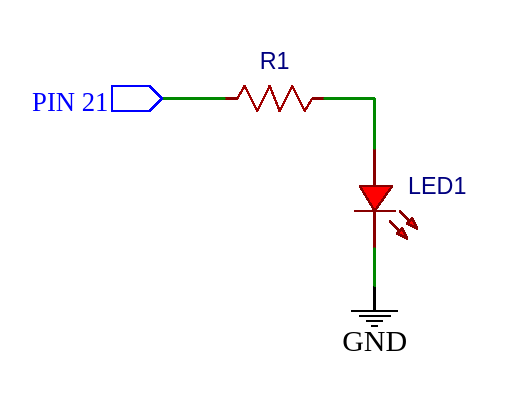# GPIO

General-Purpose Input/Output (GPIO) pins are the foundation of working with any peripherals.

In Toit, the GPIO Pin class is exposed by the `gpio` module.

GPIO pins are managed across the device. That means that only one instance of a given GPIO pin can exist at any given point in time. This ensures that two applications can't configure or use a specific GPIO pin at the same time. The pin can be released again by calling `close` after usage:

```import gpio

main:
pin := gpio.Pin 21  // Acquired system-wide.
// ...
pin.close           // Released, can be used by other applications now.```

When an application terminates, the pin will automatically be closed and released.

## Output mode

To configure a GPIO pin in output mode, either do it initially:

`  output_pin := gpio.Pin 21 --output // Initialized for output mode.`

or later (independent of previous mode):

`  pin.config --output         // Reconfigure for output mode.`

If a GPIO pin is configured for `output` mode, `Pin.set` can be used to either drive the pin voltage high (1) or low (0).

 Set value Effect `0` Low voltage `1` High voltage

### Open-drain

GPIO pins also supports output mode as open-drain. To configure the GPIO Pin as open-drain output do the following:

`  pin.config --output --open_drain`

In open-drain output mode, when setting the pin high, the pin will go into open-drain mode. That means the pin is not being pulled in any direction (floating).

 Set value Effect `0` Low voltage `1` Open drain

This can be useful when an external pull-up is connected to the pin.

## Example: LED on/off

In output-mode, the GPIO pin can be used, for example, to turn an LED on/off. The following schematic shows how a simple LED could be connected.To create a GPIO Pin on `pin 21` in output mode, use `Pin`:

```import gpio

main:
pin := gpio.Pin 21 --output```

The pin is `low (0)` by default, so to set it `high (1)` use `pin.set`.

```import gpio

main:
pin := gpio.Pin 21 --output
pin.set 1
sleep --ms=1000```

This will keep the light turned on for 1000ms.

When the program terminates, the pin will automatically be reset thus turning off the LED.

## Input mode

The GPIO input mode can be used to read the state of a pin. This can be done by calling `Pin.get`, returning either 0 or 1 depending on the voltage measured at the pin.

To configure a GPIO pin in input mode, either do it initially:

`  input_pin := gpio.Pin 21 --input // Initialized for input mode.`

or later (independent of previous mode):

`  pin.config --input         // Reconfigure for input mode.`

### Waiting for state change

While `Pin.get` can be used to get the current Pin state, sometimes it's useful to block and wait until the pin changes state. That can be done using the `Pin.wait_for` method.

```import gpio

main:
pin := gpio.Pin 21 --input
pin.wait_for 1  // Wait for pin to be high.```

### Pull-up and pull-down resistors

Some chips supports internal pull-up and/or pull-down resistors. While the size of the resistor depends on the chip used, it can be enabled like this:

`  pulled_up_pin := gpio.Pin 21 --input --pull_up`

If a Pin is configured as input with pull-up/pull-down, it automatically disables the pull-up/pull-down if it is reconfigured to output mode - and enables it again when reconfigured back to input.

The ADC (analog-to-digital converter) can be used by importing `gpio.adc`:

For example:

```import gpio

main:

Note that on the ESP32, only the ADC1 (pins 32-39) are supported. The ADC2 has too many restrictions (cannot be used when WiFi is active, and some of the pins are strapping pins), and is therefore disabled.

## PWM

The PWM (pulse width modulation) peripheral is in `pgio.pwm`.

One generally starts by allocating a `Pwm` object with a certain frequency. Multiple pins can then use that object to create their own `PwmChannel` for which they can modify the pulse width.

Example:

```import gpio
import gpio.pwm

main:
led := gpio.Pin 5
generator := pwm.Pwm --frequency=400
channel := generator.start led
duty_percent := 0
step := 1
while true:
channel.set_duty_factor duty_percent/100.0
duty_percent += step
if duty_percent == 0 or duty_percent == 100:
step = -step
sleep --ms=10```